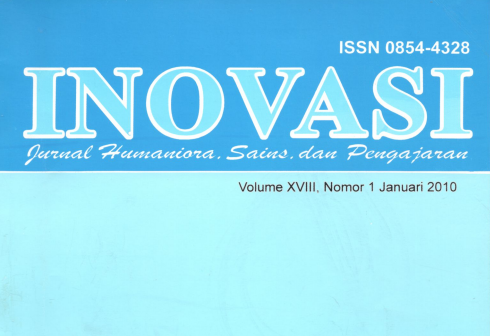(0341) 571035 library@um.ac.idArtikel Model Kompetisi Dua Spesies dari INOVASI (Jurnal Humaniora, Sains dan Pengajaran), XVIII

Model Kompetisi Dua Spesies.
Abstract:
The populations x(t) and y(t) at time t of two species that compete for the same food supply can governed with the system. Using the logistic model for the growth of a single species y, let’s assume that in the absence of species y, population x is governed by an equation of the form dx/dt = x(a1 -b1x), and, similarly, that in the absence of species x population y governed by dy/dt = y(a1 –b1y). In this paper we discuss models the evolution of the two populations in a competing species system of differential equations where both species are present and competing for the same food, the effect is to decrease their rate of growth. Generally we cannot explicitly solve the nonlinear system, however, by studying the equalitative properties of the solutions, we can obtain useful information about certain trajectories. Specially, we will try to determine how the solutions behave near a critical point. That solution of the system can be graph, so we get informations about behavior of them.
Kata kunci : kompetisi, solusi keseimbangan, titik-titik keseimbangan,bidang fase

Untuk melihat fullteks silakan klik disini

Translate »